# Śrīdhara Brāhmaṇa: QSD via SDP

Semidefinite programming (SDP) is a subfield of convex optimization concerned with the optimization of a linear objective function over the intersection of the cone of positive semidefinite matrices with an affine space, i.e., a spectrahedron.

Since January 2020, I have been providing commentary (Sanskrit ब्राह्मण, Brāhmaṇa) on mathematical pieces using every day vernacular (भाष्य, bhāṣya). Śrīdhara (श्रीधर) Brāhmaṇa (ब्राह्मण) means commentary by its author.

I first heard about SDP when Michel Goemans (Hi Michel! Sorry I missed you on my last visit to Boston in 2020) and his PhD student, David Williamson provided a slick randomized approximation for MAX-CUT (not yet improved, and it has been 25+ years) building on a SDP relaxation (an eigenvalue minimization setting, due to Delorme and Poljak) in:

Improved Approximation Algorithms for Maximum Cut and Satisfiability Problems Using Semidefinite Programming.

For a quick and easy to understand proof of this result, see the lecture notes of Ryan O’Donnell:

Switching topics, Quantum Information Theory brings together three exciting research areas of 20th Century:

Quantum Mechanics (Schrodinger, Heisenberg: 1925)

Computer Science (Turing: 1936)

Information Theory (Shannon: 1948)

In 1960s, Stratonovich, Helstrom and Gordon proposed a formulation of optical communications using quantum mechanics. This was the first historical appearance of quantum information theory. They mainly studied error probabilities and channel capacities for communication.

Quantum state discrimination (QSD) describes the distinguishability of quantum systems in different states, and the general process of extracting classical information from quantum systems. It is useful in quantum information applications, such as the characterization of mutual information in cryptographic protocols, or as a technique to derive fundamental theorems in quantum foundations. It has deep connections to physical principles such as relativistic causality.

Recall I asked in Q-AHA: Quantum Algorithms, Hardware, Applications:

What can Operations Research do for Computational Physics?

The picture on top of this post gives you pretty much what you need to get started with quantum information theory. I am serious: ket |a> is column vector and bra <b| is row vector. Multiplying a bra with a ket gives you a number (“dot product”). Multiplying a ket with a bra gives you a matrix. It is largely undergraduate linear algebra after that (with complex numbers, so refresh yourself about imaginary number and conjugates). In terms of probability, understanding finite state space takes you a long way. Sure, you also need to know Tensors, but that is just a matrix operation anyway. Fancy words like spin, Pauli matrices and entanglement, again, simply mask the simple matrix manipulations that underlie a vast majority of activity.

While much of classical information theory (Shannon, 1948) is carried over through natural quantum analogues — mutual information, relative entropy, channel capacity and so on — there are certain subtle issues in the quantum setting (conditional entropy can be negative!), and channel capacity, noise and error correction need to be handled with greater finesse. I will cover this in a future post highlighting the work of Alexander Holevo.

Now for some math to connect QSD to SDP.

The quantum analog of a probability distribution is a density operator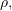a unit trace positive semi-definite matrix. Density operators provide a general way of describing the state of a quantum mechanical system.  Any measurement on a quantum system can be described mathematically using a positive operator valued measure (POVM), a set of positive semi-definite matrices that sum to the identity i.e.,Each measurement outcome occurs with probability (using Born Rule)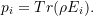A general set up for the quantum state discrimination problem is obtained as follows. Suppose a random variable X takes one of n values labeled by i with probability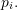When X takes on a value i, a d dimensional quantum state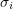is prepared that is specific to this i. The key task in quantum state discrimination is to measure the prepared state Y and predict i with high probability. That is, we wish to maximize the success probability (which does not have to be 1):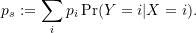Putting this all together, we get the SDP: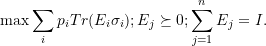Let me illustrate with a simple example that admits an algebraic solution. With arbitrary d, and n equal to 2, the SDP can be written as: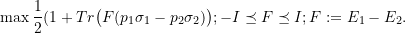This SDP has an optimum valueThe optimum value is often called the Helstrom bound.

In general, the SDP cannot be solved analytically, and numerical procedures are needed. More importantly, in most cases:

Optimal strategies of quantum state discrimination remain unsolved.

I believe Operations Research folks can contribute by bringing our expertise in SDP and approximation algorithms to this important area of quantum information theory.